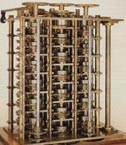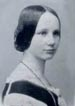Computers - Math Matters - Maplesoft

ComputersGeorge Boole (English, 1815-1864) invented Boolean algebra, the mathematical foundation of the logic used by the digital computer. In particular, computers use the special case of Boolean logic where entities can have only two values (e.g. 1 or 0, the binary system) and a set of clear rules defines their operations.

“If X is true and Y is false, or X is true and W is true, then Z is true” is a typical decision that computer programs make. In Boolean mathematical form, this decision would look like:Computers Doing Math
The idea of a machine to do mathematical calculations is not modern at all. Pascal, Leibniz, and Babbage are well-known for their attempts at automating calculations long before Microsoft®, Apple®, or Intel®.Blaise Pascal’s machine (1652) could add or subtract numbers. His most advanced models could work with numbers up to 9,999,999.Co-father of calculus Gottfried Leibniz also designed a machine (1671) that could perform addition, subtraction, multiplication and division.Charles Babbage designed Difference and Analytical Engines capable of more advanced calculations. They also featured programming through hole-punched sheets.Today, a modest machine with the right software can crunch through math that would have taken a hundred Gausses a hundred years to do. Spreadsheets help business people use math to make business decisions. Advanced software like Maple replicates the mathematical thinking that is needed to build a spacecraft or cure a disease.